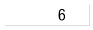# QlikView App Dev

Discussion Board for collaboration related to QlikView App Development.

cancel
Showing results for
Did you mean:Partner

## How can I keep a variable from calculating?

Hello all, sorry this may be a complicated question so I'll try to keep it as simple as possible.

I have a variable that is calculating the minimum value of a field:

v_Min_Count

=min([Count Field])

I have this value inside an Input Box so that by default, it will show the minimum value for that field, but you can enter in a static value. Since the equals sign is in the variable expression, it will always calculate the minimum. This makes it a dynamic Input Box which will react to any selections I make until a static value is put in. I have a button that will reset this variable to the expression if needed.

Part of the functionality I'm trying to create is to change the color of the text when a value has been entered, like the following

Dynamic Input Box:

=min([Count Field])Static Input Box:

10I'm doing this by adding a trigger on v_Min_Count that will set the value of another variable v_Min_Count_Changed to 1. I then go to the Text Color property on the Input Box and put: if(v_Min_Count_Changed, color1, color2)

Here is where my problem is. If I click in the Input Box, and then click out of it the variable trigger will fire and the color will change to blue even though the value is still the expression. I want the colors to represent the static nature of the value. And if the value is still the expression, then the Input Box will be dynamic and change with other selections.

My first idea was to change the text color expression to if(v_Min_Count_Changed and v_Min_Count <> '=min([Count Field])', color1, color2). But that doesn't work because v_Min_Count will be evaluated to 6.

So my question is: Is there any way that I can keep a variable with an equals sign from evaluating?

P.S. I understand that I could set the variable to be min([Count Field]) instead of =min([Count Field]) and use dollar sign expansion. The problem with doing that is that I would have to manually set the value of my Input Box to include the dollar sign and I have my variables return to default values on reload. So this isn't a possibility.

1 Solution

Accepted SolutionsMVP

Hm, you mean something like this macro returns?

Sub Test

Set ib = ActiveDocument.GetSheetObject("IB01")

rawval = ib.GetRawContent(0)

msgbox(rawval)

End sub

7 RepliesMVP

What if you compare to the expression result?

if(v_Min_Count_Changed and v_Min_Count <> min([Count Field]), color1, color2)Partner
Author

That is one possibility, but I don't think it perfectly defines the static nature I'm looking for. For instance, lets say that the field is actually [Pct Field] and has a range of 0-100%. If a 0 was entered into the input box, it would become static but would still be colored black because it is still equal to the min of [Pct Field].Partner
Author

Perhaps another way I can describe it is that I would like to do the opposite of dollar sign expansion. So instead of calculating the expression I would like to get the expression text. Is this possible?MVP

Hm, you mean something like this macro returns?

Sub Test

Set ib = ActiveDocument.GetSheetObject("IB01")

rawval = ib.GetRawContent(0)

msgbox(rawval)

End subPartner
Author

YES! That is exactly what I'm looking to return. I haven't used macros that much, but I'm sure that I can figure it out from there. Thanks for all the help!MVP

Danny, attached is a sample QVW.Partner
Author

Awesome! Thanks again!